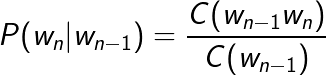## Python Tutorials: Your guide to Natural Language Processing in Python

##### Python Tutorials: In this part of Learning Python we Cover Natural Language Processing In Python
Written by |20-Oct-2020 | 0 Comments | 650 Views

Minimum Edit Distance:

In computer sciences, edit distance is a way of calculating how dissimilar two strings are from each other by counting the minimum edit distance by the minimum operations required to perform one string into another.

Edit distance finds applications in natural language processing where programmed spelling amendment can decide candidate corrections for an incorrectly spelled word by choosing words from a dictionary that have a lesser distance to the word in question.

In bioinformatics, it very well may be utilized to measure the similarity between two DNA sequences. Which are the sequence of A, G, T, and H. This technique is used in finding whether the person has thalassemia or not?

The minimum edit distance is calculated through dynamic programming. Suppose we have two strings, "abcdef" and "azced."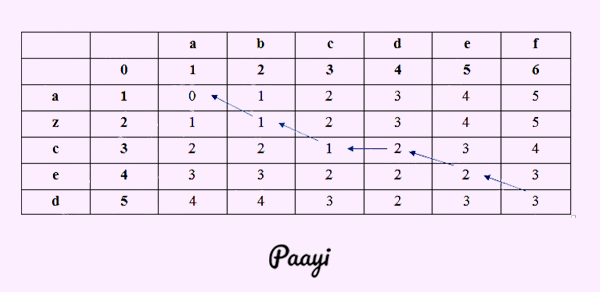Figure 0

The minimum distance between the two strings is 3.

The blue arrows show the backtrace. Backtrace will elaborate more about the minimum distance. By this backtrace, we can say that there are two substitutions and one deletion.

Programmatically we can write it as:## Figure 1

Why Backtrace?

Edit distance is not sufficient, and we often need to align each character of the two strings to one another. We do this by keeping a backtrace. Each time we enter a cell, remember where we originated from when we attain the end—Traceback the path from the upper right corner to analyze the alignment.

This traceback will tell us how many insertion-deletion and substitution occurred.

Why Sequence Alignment?

• Comparing genes or region from different species

• to find the vital region, determine their functionality, and uncover evolutionary forces.
• We are assembling fragments to sequence DNA.
• Compare individuals to looking for mutations.

In natural language processing, we generally describe the minimum distances and weights just in the case of a spelling check. In the case of computational biology, we generally talk about the similarity and scores.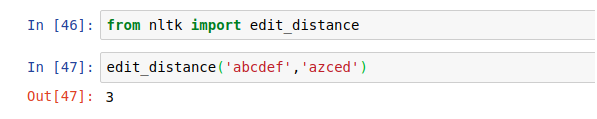## N-Grams:

Models that assign probabilities to a sequence of words are called language models. An n-gram is a sequence of the text of n-word: a bigram is a two-word sequence of a word like "please turn," "turn your," and "your homework." Similarly, a trigram has three words of sequence.

The n-grams assign the probability to each sequence and then compute what the probability of that sequence after a particular sequence is. Whether estimating probabilities of the next word or whole sequences, the N-gram model is one of the essential tools in speech and language processing.

Let's begin with the task of computing P(w|h), the probability of a word w given some history h: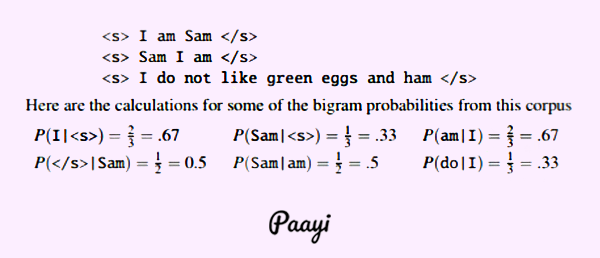Figure 3

From the above example, we can say that the probability of "am" after I am high and the probability of "am" after "Sam" is high.

Introduction to N-Gram:

Models that assign probabilities to a sequence of words are called language models. An N-Gram is known as a sequence of N words: a bigram is a two-word sequence of a word like "please turn," "turn your," and "your homework." Similarly, a trigram has three words of sequence.

The n-grams assign the probability to each sequence and then compute what the probability of that sequence after a particular sequence is. Whether estimating probabilities of the next word or whole sequences, the N-gram model is one of the essential tools in speech and language processing.

• N-Grams are sequences of tokens.
• The N stands for how many terms are used. It can be a unigram that is one term, a bigram with two terms, and a trigram with three terms.

We can use different types of tokenization for this and that are Character-based n-grams, words based n-grams, and Part of speech-based n-grams. The n-grams give us a background idea of the context around the token we are looking at.

N-gram is a language model is a model that lets us compute the probability, or likelihood of a sentence S, P(S). It uses the previous N-1 words in a sequence to predict the next word. Then we train these language models by counting the frequency distribution in large corpora.

Let's start with the assignment of computing P(w|h), the likelihood of a word w given some history h. Assume the history "h" is "its water is so transparent that" and we need to know the probability that the following word is the:

P( the | its water is transparent that )

the method of estimation of the sentence is: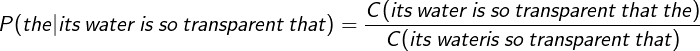Similarly, if we want to know the joint probability of an entire sequence of a word like its water is so transparent, we could do it by asking out of all possible sequences of five words how many of them are its water is so transparent that. That is a lot to estimates.

A smart way to find the probability is by using the chain rule of probability: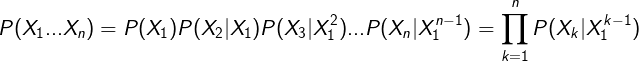Applying the chain rule, we get: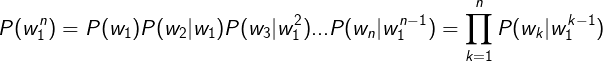The chain rule displays the connection between computing the joint probability of a sequence and figuring the conditional probability of a word given. The equation quoted above discloses to us that we can evaluate the joint probability of a whole series of words by multiplying together several conditional probabilities.

The intuition of N-Gram:

The intuition behind the n-gram model is that instead of computing the likelihood of a word given its whole history, we can estimate the history by merely the last couple of words. When we utilize a bigram model to predict the conditional probability of the following word, we are consequently making the following estimate:It is based on the Markov assumption. Markov models are the class of probabilistic models that assume we can predict the probability without looking too far into the past.

An intuitive way to estimate probabilities of bigram or n-gram is called maximum likelihood estimation or MLE. We get the MLE estimates for the parameters of an n-gram model by getting counts from a corpus and normalize the count so that they lie between 0 and 1.

For instance, to compute a specific bigram probability of a word y given previous word x, we'll compute the count of bigram and normalize by the sum of all the bigrams and share the same word x: